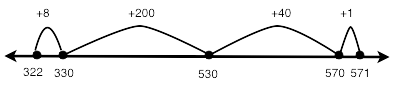Author:
DAWNE COKER
Subject:
Mathematics
Material Type:
Reference Material, Teaching/Learning Strategy
Level:
Lower Primary
Tags:
• 2-digit
• 3-digit
• Approach
• Break Apart
• Cluster 4
• Cluster 5
• Compose
• Decompose
• Number Line
• Place Value
• Strategy
• Subtract
• Subtraction
• Tool
• Unit 4
• Unit 5
Language:
English
Media Formats:

# T4T Strategies for Adding & Subtracting 3-Digit Numbers## Overview

This resoruce is from Tools4NCTeachers.

Second graders use a variety of strategies based on place value and number relationships to add and subtract multi-digit numbers.  The first attachment in this resource outlines strategies for adding 3-digit numbers, and the second attachment outlines strategies for subtracting 3-digit numbers.

Second graders are not taught the standard algorithm.

Here is a sample of this resource. Click the attachments to access the fully-formatted files.

Strategies for Solving Three-Digit Addition Problems

These are examples of strategies students could use to solve 3-digit problems.  These are not the only strategies students could use.

Counting Up

322 + 249                                                                    322 + __________ = 571

322 + 200 = 522

522 + 40 = 562                                                             322 + 8 = 330

562 + 9 = 571                                                              330 + 70 = 400 (or the student could count up by 7 tens)

400 + 100 = 500

500 + 71 = 571322

422                 522            532 542 552 562    563, 564, 565, …… 571

Place Value

322 + 249                                                                                                                      322

300 + 200 = 500                                                                                                           +249

20 + 40 = 60                                                                                                                  500

2 + 9 = 11                                                                                                                       60

500 + 60 + 11 = 571                                                                                                     +  11

57